Support the Monkey! Tell All your Friends and TeachersHome MonkeyNotes Printable Notes Digital Library Study Guides Study Smart Parents Tips College Planning Test Prep Fun Zone Help / FAQ How to Cite New Title Request

 Section 7: Mathematical Reasoning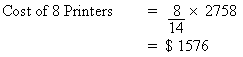Ans : B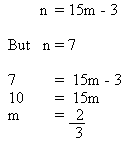Ans : C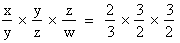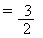Ans : B S1 = 1.25S where S1 = New side of square                               S = Old side of square S12 = (1.25S)2 S12 = 1.5625S2 \ New area is 1.5625S2 - S2           = 0.5625S2 more than old square           = 56.25% more Ans : D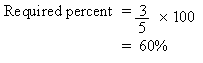Ans : B Let the number be x.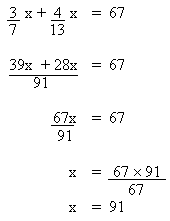Ans : D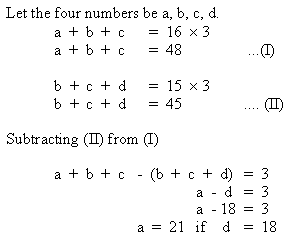Ans : D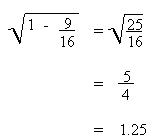Ans : D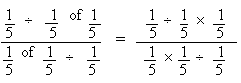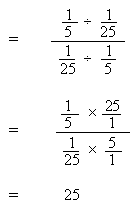Ans : A Index Test 3 Section 1: Verbal Reasoning Section 2: Mathematical Reasoning Section 3: Verbal Reasoning Section 4: Mathematical Reasoning Section 5: Reading Comprehension Section 6: Mathematical Reasoning Section 7: Mathematical Reasoning Answer Key To Test 3 Answer Explanation To Test 3 Section 1: Verbal Reasoning Section 2: Mathematical Reasoning Section 3: Verbal Reasoning Section 4: Mathematical Reasoning Section 5: Reading Comprehension Section 6: Mathematical Reasoning Section 7: Mathematical Reasoning Test 4
 Search: All Products Books Popular Music Classical Music Video DVD Toys & Games Electronics Software Tools & Hardware Outdoor Living Kitchen & Housewares Camera & Photo Cell Phones Keywords: